oomph::IsotropicStrainEnergyFunctionConstitutiveLaw Class Reference

////////////////////////////////////////////////////////////////////// ////////////////////////////////////////////////////////////////////// More...

#include <constitutive_laws.h>Inheritance diagram for oomph::IsotropicStrainEnergyFunctionConstitutiveLaw:

## Public Member Functions

IsotropicStrainEnergyFunctionConstitutiveLaw (StrainEnergyFunction *const &strain_energy_function_pt)
Constructor takes a pointer to the strain energy function. More...

void calculate_second_piola_kirchhoff_stress (const DenseMatrix< double > &g, const DenseMatrix< double > &G, DenseMatrix< double > &sigma)
Calculate the contravariant 2nd Piola Kirchhoff stress tensor. Arguments are the covariant undeformed and deformed metric tensor and the matrix in which to return the stress tensor. Uses correct 3D invariants for 2D (plane strain) problems. More...

void calculate_second_piola_kirchhoff_stress (const DenseMatrix< double > &g, const DenseMatrix< double > &G, DenseMatrix< double > &sigma_dev, DenseMatrix< double > &G_contra, double &Gdet)
Calculate the deviatoric part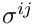of the contravariant 2nd Piola Kirchhoff stress tensor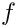. Also return the contravariant deformed metric tensor and the determinant of the deformed metric tensor. This form is appropriate for truly-incompressible materials for which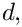where the "pressure"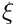is determined by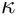. More...

void calculate_second_piola_kirchhoff_stress (const DenseMatrix< double > &g, const DenseMatrix< double > &G, DenseMatrix< double > &sigma_dev, DenseMatrix< double > &Gcontra, double &gen_dil, double &inv_kappa)
Calculate the deviatoric part of the contravariant 2nd Piola Kirchoff stress tensor. Also return the contravariant deformed metric tensor, the generalised dilatation,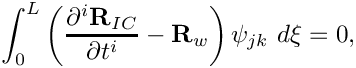and the inverse of the bulk modulus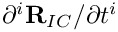. This form is appropriate for near-incompressible materials for which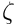where the "pressure"is determined from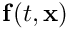. More...

bool requires_incompressibility_constraint ()
State if the constitutive equation requires an incompressible formulation in which the volume constraint is enforced explicitly. Used as a sanity check in PARANOID mode. This is determined by interrogating the associated strain energy function. More...Public Member Functions inherited from oomph::ConstitutiveLaw
ConstitutiveLaw ()
Empty constructor. More...

virtual ~ConstitutiveLaw ()
Empty virtual destructor. More...

virtual void calculate_d_second_piola_kirchhoff_stress_dG (const DenseMatrix< double > &g, const DenseMatrix< double > &G, const DenseMatrix< double > &sigma, RankFourTensor< double > &d_sigma_dG, const bool &symmetrize_tensor=true)
Calculate the derivatives of the contravariant 2nd Piola Kirchhoff stress tensor with respect to the deformed metric tensor. Arguments are the covariant undeformed and deformed metric tensor, the current value of the stress tensor and the rank four tensor in which to return the derivatives of the stress tensor The default implementation uses finite differences, but can be overloaded for constitutive laws in which an analytic formulation is possible. If the boolean flag symmetrize_tensor is false, only the "upper triangular" entries of the tensor will be filled in. This is a useful efficiency when using the derivatives in Jacobian calculations. More...

virtual void calculate_d_second_piola_kirchhoff_stress_dG (const DenseMatrix< double > &g, const DenseMatrix< double > &G, const DenseMatrix< double > &sigma, const double &detG, const double &interpolated_solid_p, RankFourTensor< double > &d_sigma_dG, DenseMatrix< double > &d_detG_dG, const bool &symmetrize_tensor=true)
Calculate the derivatives of the contravariant 2nd Piola Kirchhoff stress tensor. with respect to the deformed metric tensor. Also return the derivatives of the determinant of the deformed metric tensor with respect to the deformed metric tensor. This form is appropriate for truly-incompressible materials. The default implementation uses finite differences for the derivatives that depend on the constitutive law, but not for the derivatives of the determinant, which are generic. / If the boolean flag symmetrize_tensor is false, only the "upper triangular" entries of the tensor will be filled in. This is a useful efficiency when using the derivatives in Jacobian calculations. More...

virtual void calculate_d_second_piola_kirchhoff_stress_dG (const DenseMatrix< double > &g, const DenseMatrix< double > &G, const DenseMatrix< double > &sigma, const double &gen_dil, const double &inv_kappa, const double &interpolated_solid_p, RankFourTensor< double > &d_sigma_dG, DenseMatrix< double > &d_gen_dil_dG, const bool &symmetrize_tensor=true)
Calculate the derivatives of the contravariant 2nd Piola Kirchoff stress tensor with respect to the deformed metric tensor. Also return the derivatives of the generalised dilatation,with respect to the deformed metric tensor. This form is appropriate for near-incompressible materials. The default implementation uses finite differences. If the boolean flag symmetrize_tensor is false, only the "upper triangular" entries of the tensor will be filled in. This is a useful efficiency when using the derivatives in Jacobian calculations. More...

## Private Attributes

StrainEnergyFunctionStrain_energy_function_pt
Pointer to the strain energy function. More...Protected Member Functions inherited from oomph::ConstitutiveLaw
bool is_matrix_square (const DenseMatrix< double > &M)
Test whether a matrix is square. More...

bool are_matrices_of_equal_dimensions (const DenseMatrix< double > &M1, const DenseMatrix< double > &M2)
Test whether two matrices are of equal dimensions. More...

void error_checking_in_input (const DenseMatrix< double > &g, const DenseMatrix< double > &G, DenseMatrix< double > &sigma)
Check for errors in the input, i.e. check that the dimensions of the arrays are all consistent. More...

double calculate_contravariant (const DenseMatrix< double > &Gcov, DenseMatrix< double > &Gcontra)
Calculate a contravariant tensor from a covariant tensor, and return the determinant of the covariant tensor. More...

void calculate_d_contravariant_dG (const DenseMatrix< double > &Gcov, RankFourTensor< double > &dGcontra_dG, DenseMatrix< double > &d_detG_dG)
Calculate the derivatives of the contravariant tensor and the derivatives of the determinant of the covariant tensor with respect to the components of the covariant tensor. More...

## Detailed Description

////////////////////////////////////////////////////////////////////// //////////////////////////////////////////////////////////////////////

A class for constitutive laws derived from strain-energy functions. Theory is in Green and Zerna.

Definition at line 800 of file constitutive_laws.h.

## ◆ IsotropicStrainEnergyFunctionConstitutiveLaw()

 oomph::IsotropicStrainEnergyFunctionConstitutiveLaw::IsotropicStrainEnergyFunctionConstitutiveLaw ( StrainEnergyFunction *const & strain_energy_function_pt )
inline

Constructor takes a pointer to the strain energy function.

Definition at line 808 of file constitutive_laws.h.

## ◆ calculate_second_piola_kirchhoff_stress() [1/3]

 void oomph::IsotropicStrainEnergyFunctionConstitutiveLaw::calculate_second_piola_kirchhoff_stress ( const DenseMatrix< double > & g, const DenseMatrix< double > & G, DenseMatrix< double > & sigma )
virtual

Calculate the contravariant 2nd Piola Kirchhoff stress tensor. Arguments are the covariant undeformed and deformed metric tensor and the matrix in which to return the stress tensor. Uses correct 3D invariants for 2D (plane strain) problems.

////////////////////////////////////////////////////////////////////// //////////////////////////////////////////////////////////////////////

Calculate the contravariant 2nd Piola Kirchhoff stress tensor. Arguments are the covariant undeformed and deformed metric tensor and the matrix in which to return the stress tensor. Uses correct 3D invariants for 2D (plane strain) problems.

Implements oomph::ConstitutiveLaw.

Definition at line 895 of file constitutive_laws.cc.

## ◆ calculate_second_piola_kirchhoff_stress() [2/3]

 void oomph::IsotropicStrainEnergyFunctionConstitutiveLaw::calculate_second_piola_kirchhoff_stress ( const DenseMatrix< double > & g, const DenseMatrix< double > & G, DenseMatrix< double > & sigma_dev, DenseMatrix< double > & G_contra, double & Gdet )
virtual

Calculate the deviatoric partof the contravariant 2nd Piola Kirchhoff stress tensor. Also return the contravariant deformed metric tensor and the determinant of the deformed metric tensor. This form is appropriate for truly-incompressible materials for whichwhere the "pressure"is determined by.

Calculate the deviatoric partof the contravariant 2nd Piola Kirchhoff stress tensor. Also return the contravariant deformed metric tensor and the determinant of the deformed metric tensor. Uses correct 3D invariants for 2D (plane strain) problems. This is the version for the pure incompressible formulation.

Reimplemented from oomph::ConstitutiveLaw.

Definition at line 1009 of file constitutive_laws.cc.

## ◆ calculate_second_piola_kirchhoff_stress() [3/3]

 void oomph::IsotropicStrainEnergyFunctionConstitutiveLaw::calculate_second_piola_kirchhoff_stress ( const DenseMatrix< double > & g, const DenseMatrix< double > & G, DenseMatrix< double > & sigma_dev, DenseMatrix< double > & Gcontra, double & gen_dil, double & inv_kappa )
virtual

Calculate the deviatoric part of the contravariant 2nd Piola Kirchoff stress tensor. Also return the contravariant deformed metric tensor, the generalised dilatation,and the inverse of the bulk modulus. This form is appropriate for near-incompressible materials for whichwhere the "pressure"is determined from.

Calculate the deviatoric part of the contravariant 2nd Piola Kirchoff stress tensor. Also return the contravariant deformed metric tensor, the generalised dilatation,and the inverse of the bulk modulus. Uses correct 3D invariants for 2D (plane strain) problems. This is the version for the near-incompressible formulation.

Reimplemented from oomph::ConstitutiveLaw.

Definition at line 1136 of file constitutive_laws.cc.

## ◆ requires_incompressibility_constraint()

 bool oomph::IsotropicStrainEnergyFunctionConstitutiveLaw::requires_incompressibility_constraint ( )
inlinevirtual

State if the constitutive equation requires an incompressible formulation in which the volume constraint is enforced explicitly. Used as a sanity check in PARANOID mode. This is determined by interrogating the associated strain energy function.

Implements oomph::ConstitutiveLaw.

Definition at line 861 of file constitutive_laws.h.

## ◆ Strain_energy_function_pt

 StrainEnergyFunction* oomph::IsotropicStrainEnergyFunctionConstitutiveLaw::Strain_energy_function_pt
private

Pointer to the strain energy function.

Definition at line 804 of file constitutive_laws.h.

The documentation for this class was generated from the following files: